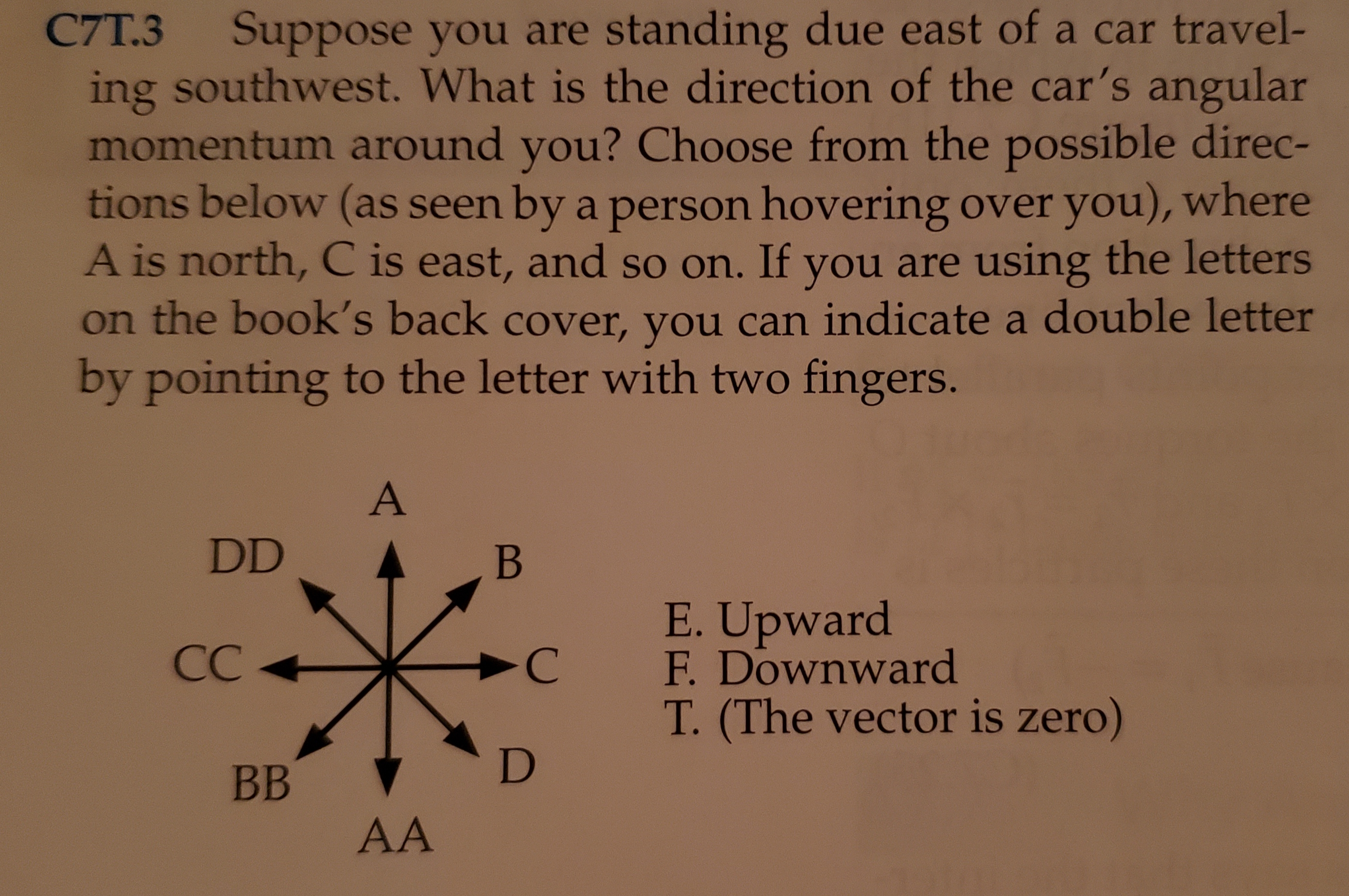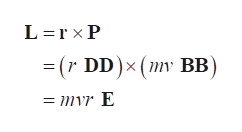C7T.3Suppose you are standing due east of a car travel-ing southwest. What is the direction of the car's angularmomentum around you? Choose from the possible direc-tions below (as seen by a person hovering over you), whereA is north, C is east, and so on. If you are using the letterson the book's back cover, you can indicate a double letterby pointing to the letter with two fingers.ADDВE.UpwardF. DownwardСС-CT. (The vector is zero)DВBAA

Questionhelp_outlineImage TranscriptioncloseC7T.3 Suppose you are standing due east of a car travel- ing southwest. What is the direction of the car's angular momentum around you? Choose from the possible direc- tions below (as seen by a person hovering over you), where A is north, C is east, and so on. If you are using the letters on the book's back cover, you can indicate a double letter by pointing to the letter with two fingers. A DD В E.Upward F. Downward СС -C T. (The vector is zero) D ВB AA fullscreen
Step 1

Consider the direction diagram given in the question.

As the car is travelling south-west, car’s velocity direction can be written as below. Let v be the magnitude of velocity. Write the vector direction according to the direction diagram.

Step 2

Also, the person is standing due east of the car. Write the position vector of the person with respect to the origin.

Step 3

Write the expression of angular momentum about a point in vector form. Let m...help_outlineImage TranscriptioncloseL r x P ( DD)x ( BB) = mvr E fullscreen

Want to see the full answer?

See Solution

Want to see this answer and more?

Our solutions are written by experts, many with advanced degrees, and available 24/7

See Solution
Tagged in

Angular Motion